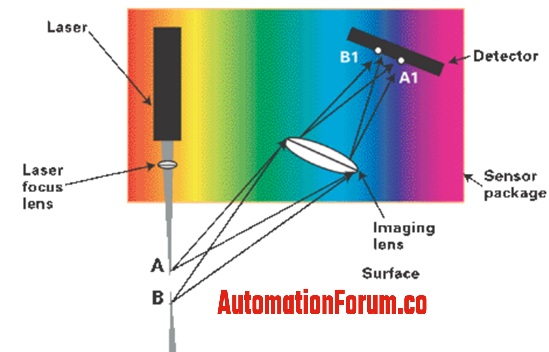Displacement Measurement

# What is triangulation and explain triangulation sensor?Triangulation is a method that is used to determine the distance by using angles and trigonometry. In a triangulation type of distance measurement, the light will be transmitted at a known angle from the transmitter and the receiver would detect the light direction which is received. So according to the angle at which the light is received the distance is calculated. The triangulation principle is used for the short distance measurements, in the range with high resolution. The triangulation sensor would measure the distance of a target from the image data using the sensor’s microprocessor. So basically this type of distance sensor would detect the target distance with the help of sensor and source angle.

### What is the need for a laser triangulation sensor and where do we use it?

These sensors are capable to measure the distance to small fragile parts of soft surfaces that would badly affect by physical contact. So it would need a non-contact type of sensor, so during this case, we can use a laser triangulation sensor and this won’t use a probe to do the measurement. This sensor would use a light source to do the measurement and the light will be transmitted to the object surface that is to be measured and this light source will be reflected by a lens.

A triangulation sensor can work on most type of the light source, in order to get a good performance the light source should be of high intensity and it needs to create a small spot on the surface. Due to this requirement, all the triangulation sensors would use a laser as a light source.

### How does a laser triangulation sensor works?

By using a triangulation sensor we can do the displacement measurement accurately, with the help of a proper receiver and position sensitive detector this sensor can determine the distance of the object. So basically in this sensor, there would be a light source, so a laser would project a beam of light onto the surface that is to be measured. The operation of this sensor is based on the reflection of light from a source to a position sensing detector. So mostly all the materials are optically a combination of diffuse and spectacular surfaces and it is because a change in reflectivity would change the level of intensity of the light reaching the detector.

The operation of this sensor is shown in the above image, the laser would project a spot of light at position A. A lens is used at a certain angle from the laser beam in order to create an image of this spot at position A1. The surface spot will be in position B if the surface is not near to the sensor and the spot location would change to B1. By determining the position of the image spot and also by calculating the angle we can determine the distance from the sensor to the target.

### What are the advantages of non-contact displacement measurement?

The non-contact measurement is very useful because it doesn’t make any contact with the object that is to be measured and these sensors are free of probe wear and bounce, it would collect data at higher rates than contact devices and it won’t influence the object that is being measured.

### What are the advantages of triangulation sensors?

• We can detect objects that are fragile or substances that could easily be damaged
• This device can be used for the in-process checks of the surfaces that are wet or soft such as sealants, inks, or solder pastes.
• It is used to check the dynamic materials
• In order to do the vibration monitoring, we can use laser triangulation sensors
• The distance from the sensor to the object is better than the capacitive and inductive sensors
• These sensors have good resolution and accuracy when compared to an ultrasonic sensor